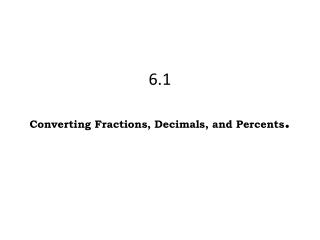DownloadDownload Presentation6.1 Converting Fractions , Decimals , and Percents .

# 6.1 Converting Fractions , Decimals , and Percents .

Télécharger la présentation## 6.1 Converting Fractions , Decimals , and Percents .

- - - - - - - - - - - - - - - - - - - - - - - - - - - E N D - - - - - - - - - - - - - - - - - - - - - - - - - - -
##### Presentation Transcript

1. 6.1Converting Fractions, Decimals, and Percents.

2. 6.1 Pg 14 • Percent -Percent means “per 100” or how many “out of 100”; percent is another name for hundredths. • Decimal-A numerical value less than 1. For example, 0.875 is a decimal. • Equivalent -Expressions that have the same value or that have the same mathematical meaning. For example, ½ and 0.5 are equivalent. • Fraction-A number representing some part of a whole or part of a set; one number compared to another in the form a/b. • Hundredths -A numerical value two decimal places to the right of a decimal point. The hundredths place for the numerical value 3.458 would be 5. • tenths-A numerical value one decimal place to the right of a decimal point. The tenths place for the numerical value 0.25 would be 2. • 10 x 10 grid -A grid of 100 units used to represent percents Pg 13 Directions: Fraction to decimal- Divide the numerator by the denominator Decimal to percent- Move the decimal point two spaces to the right Percent to fraction -denominator is 100, then simplify Percent to decimal- move the decimal point two spaces to the left Decimal to fraction- Use place value ._ _ _ (tenths, hundredths, thousandths) Foldable FRACTION DECIMAL PERCENT ¼ .25 25% ½ .5 50% ¾ .75 75% 4/4 1 100% 1/5 .2 20% 2/5 .4 40% 3/5 .6 60% 4/5 .8 80% 5/5 1 100% 1/8 .125 12.5% 2/8= ¼ .25 25%